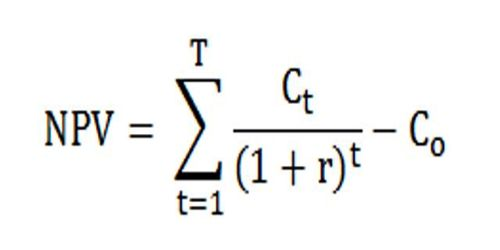# Possibilities of Net Present Value (NPV)

Possibilities of Net Present Value (NPV) –

Net present value is the difference between the present value of cash inflows and the present value of cash outflows that occur as a result of undertaking an investment project. It may be positive, zero or negative. A positive NPV means the investment is worthwhile, an NPV of 0 means the inflows equal the outflows, and a negative NPV means the investment is not good for the investor. These three possibilities of net present value are briefly explained below:

Positive NPV: If the present value of cash inflows is greater than the present value of the cash outflows, the net present value is said to be positive and the investment proposal is considered to be acceptable. If NPV is positive then it means you’re paying less than what the asset is worth.

Zero NPV: If the present value of cash inflow is equal to the present value of cash outflow, the net present value is said to be zero and the investment proposal is considered to be acceptable. If NPV is zero then it means you’re paying exactly what the asset is worth.

Negative NPV: If the present value of cash inflow is less than the present value of cash outflow, the net present value is said to be negative and the investment proposal is rejected. If NPV is negative then it means that you’re paying more than what the asset is worth.